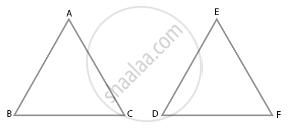# It is given that ∆ABC ~ ∆EDF such that AB = 5 cm, AC = 7 cm, DF = 15 cm and DE = 12 cm. Find the lengths of the remaining sides of the triangles. - Mathematics

Sum

It is given that ∆ABC ~ ∆EDF such that AB = 5 cm, AC = 7 cm, DF = 15 cm and DE = 12 cm. Find the lengths of the remaining sides of the triangles.

#### SolutionGiven,

∆ABC ∼ ∆EDF

From property of similar triangle,

We know that, corresponding sides of ∆ABC and ∆EDF are in the same ratio.

(AB)/(ED) = (AC)/(EF) = (BC)/(DF)   .......(i)

AB = 5cm, AC = 7cm

DF = 15cm and DE = 12cm

Substituting these values in equation (i), we get,

5/12 = 7/(EF) = (BC)/15

On taking 5/12 = 7/(EF), we get,

5/12 = 7/(EF)

EF = (12 xx 7)/5 = 16.8 cm

On taking 5/12 = (BC)/15, we get,

5/12 = (BC)/15

BC = (5 xx 15)/12 = 6.25 cm

Hence, lengths of the remaining sides of the triangles are EF = 16.8 cm and BC = 6.25 cm

Concept: Similarity of Triangles
Is there an error in this question or solution?

#### APPEARS IN

NCERT Mathematics Exemplar Class 10
Chapter 6 Triangles
Exercise 6.4 | Q 2 | Page 73

Share